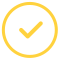effortlessly.

# What is Diagonalization of a Matrix?

Matrix diagonalization refers to the process of taking a square matrix and converting it into a particular type of matrix (diagonal matrix) that borrows from the same fundamental properties of the underlying matrix. It equates to transforming the underlying system of equations into a unique set of coordinate axes in which the matrix takes this canonical form.

When we diagonalize a matrix, we try to find the matrix's eigenvalues, which turn out to be the diagonalized matrix's precise entries. The eigenvectors similarly make up the new set of axes, which correspond to the diagonal matrix.

Diagonalization of a matrix calculator does all this for you in a split of a second.

## How To Find Diagonalization of Matrix

Finding the diagonalization of a matrix goes in tandem with the three values described above:

• a diagonalized matrix,
• eigenvalues, and
• eigenvectors

Now, a square matrix A can be decomposed into the following special form:

A=PDP^ (-1),

Where,

P = the matrix composed of the eigenvectors of A,

D = the diagonal matrix constructed from the corresponding eigenvalues, and

P^ (-1) =

the matrix inverse of P

An initial matrix theorem according to the Eigen decomposition theorem is as follows:

AX = Y

It can be written as:

PDP^ (-1) X=Y

By pre-multiplying both sides by P^ (-1), we get:

DP^ (-1) X=P^ (-1) Y.

Therefore,

DX^'=Y^'

Where,

X^'=P^(-1)X and

Y^'=P^ (-1) Y

Using a linear algebra matrix diagonalization calculator, you can reach your results by keying in the values. The above function works for a diagonalizable matrix.

Even when you have a rotational matrix that is not diagonalizable over the reals, it is always possible to find a matrix with similar properties, made up of eigenvalues on the leading diagonal. If this task seems complicated to you, you can always use math writing to get out of the mud.

The procedure of a matrix diagonalization is as follows:

• First, find the characteristic polynomial
• Move on to find the eigenvalues
• Then find the Eigen spaces
• Determine the linearly independent eigenvectors
• Define the invertible matrix
• Define the diagonal matrix
• Finish the diagonalization

Check out the following sample problem and solution:

Consider the $2\times 2$ complex matrix

$A=\begin{bmatrix} a & b-a\\ 0& b \end{bmatrix}.$

To find the eigenvalues of $A$:

Since $A$ is an upper triangular matrix, eigenvalues are diagonal entries.

Hence $a, b$ are eigenvalues of $A$.

Math experts can give you a step by procedure on how to complete the problem to arrive at the Eigenvectors.

### How To Use The Diagonalize Matrix Calculator

Before opting to get help with math homework, you can effortlessly find your answers on the Diagonalize matrix calculator through the following steps:

First, choose the dimensions of the matrix

And finally, enter the elements of the matrix

You will get your answer in the blink of an eye rather than manually having to compute it all by yourself.

### Math Experts To Do Your Homework

If the calculator does not compute something for you or feel dissatisfied with the answer, you can use the 'pay someone to do my math homework' option. You will get quality, cheap, and fast online help.

Let’s make your assignment go away.Awesome. Now you'll never miss out.